# Core Plot Tutorial: Getting Started

In this Core Plot tutorial you’ll learn how to plot your data on beautiful pie and scatter charts using Swift and the open source Core Plot framework.

5/5 2 Ratings

#### Version

• Swift 3, iOS 10, Xcode 8

Note: This Core Plot tutorial has been updated for iOS 10 and Swift 3.0 by Attila Hegedüs. The original tutorial was written by tutorial team member Steve Baranski.

If you’ve ever wanted to include charts or graphs in your app, chances are you’ve considered the following two options:

1. DIY. Write all of the drawing code yourself using Core Graphics and/or Quartz. However, this can be a lot of work.
2. Buy it! Purchase a commercial framework like ShinobiControls. This may save you time, but it will cost you to use it.

But what if you don’t want to the spend time and effort to write something from scratch, yet you also don’t want to shell out a ton of money? That’s where a third option comes in handy: use the open-source Core Plot library!

Core Plot is a 2D plotting library for iOS, Mac OS X, and tvOS. It uses Apple frameworks like Quartz and Core Animation; it has solid test coverage; and it’s released under a permissive BSD license.

In this Core Plot tutorial, you will learn how to use Core Plot to create pie charts and bar graphs. You’ll also create cool chart interactions!

Before you begin, you need to have Xcode 8.0 installed and a basic understanding of Swift, Interface Builder and storyboards. If you are new to any of these topics, you should go through some of our other tutorials first before continuing with this Core Plot tutorial.

This Core Plot tutorial also uses CocoaPods to install third-party library dependencies. If you’ve never used CocoaPods before, you should read our tutorial about it first.

## Getting Started

In this Core Plot tutorial, you’ll create an app that displays currency exchange rates for a given time interval. Download the starter project for this Core Plot tutorial from here. Unzip the archive, and open SwiftRates.xcworkspace.

The key classes for this Core Plot tutorial are located under the App group and its subgroups. These include:

• DataStore.swift
This is a helper class that requests currency exchange rates from Fixer.io.
• Rate.swift
This is a model representing currency exchange rates on a given date.
• Currency.swift
This is a model for a currency type. The supported currencies are defined in Resources/Currencies.plist.
This is the first view controller shown when the app launches. It lets the user select a base currency and two comparisons.
• HostViewController.swift
This is a container view controller that displays either `PieChartViewController` or `BarGraphViewController` based on its segmented control’s selected index. It also takes care of requesting rates from the `DataStore`, which it sets on its displayed view controller.
• PieChartViewController.swift
This will show a pie chart for exchange rates on a given day. You’ll implement this chart first!
• BarGraphViewController.swift
This will show a bar graph for exchange rates over several days. After mastering the pie chart, this will be a piece of cake! (See what I did there? Oh, come on! It was a little funny.) ;]

Build and run to see the starter project for this Core Plot tutorial in action.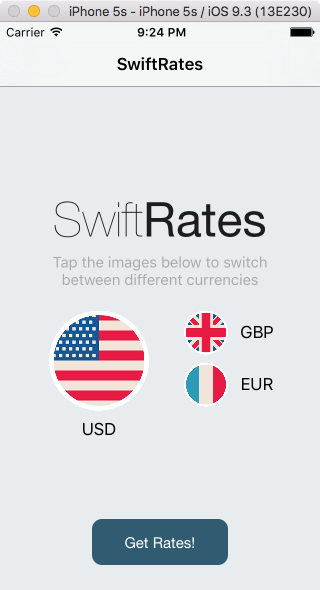Select Get Rates to navigate to the `HostViewController` and then change the segmented control’s selection. The app really doesn’t do very much… yet. ;]

It’s time in this Core Plot tutorial to get plotting!

### Installing Core Plot

First in this Core Plot tutorial, you need to install Core Plot. The easiest way to do this is via CocoaPods.

Add the following to your Podfile, right after the `pod 'SwiftDate'` line:

```pod 'CorePlot', '~> 2.2'
```

Open Terminal; `cd` into your project directory; and run `pod install`.

After the install completes, build the project.

No errors, right? Great, you’re all setup to use Core Plot. Thanks, CocoaPods. :]

If you do get any errors, try updating CocoaPods via `sudo gem install cocoapods` and then `pod install` again.

## Creating the Pie Chart

Open PieChartViewController.swift and add the following import:

```import CorePlot
```

```@IBOutlet weak var hostView: CPTGraphHostingView!
```

`CPTGraphHostingView` is responsible for “hosting” a chart/graph. You can think of it as a “graph container”.

Next, add the following class extension after the ending class curly brace:

```extension PieChartViewController: CPTPieChartDataSource, CPTPieChartDelegate {

func numberOfRecords(for plot: CPTPlot) -> UInt {
return 0
}

func number(for plot: CPTPlot, field fieldEnum: UInt, record idx: UInt) -> Any? {
return 0
}

func dataLabel(for plot: CPTPlot, record idx: UInt) -> CPTLayer? {
return nil
}

func sliceFill(for pieChart: CPTPieChart, record idx: UInt) -> CPTFill? {
return nil
}

func legendTitle(for pieChart: CPTPieChart, record idx: UInt) -> String? {
return nil
}
}
```

You provide data for a Core Plot chart via `CPTPieChartDataSource`, and you get user interaction events via `CPTPieChartDelegate`. You’ll fill in these methods as the Core Plot tutorial progresses.

### Setting Up the Graph Host View

To continue this Core Plot tutorial, open Main.storyboard and select the `PieChartViewController` scene.

Drag a new `UIView` onto this view. Change its class to `CPTGraphHostingView`, and connect it to the `hostView` outlet.

Add constraints on each side to pin this view to its parent view, making sure that Constrain to margins is NOT set: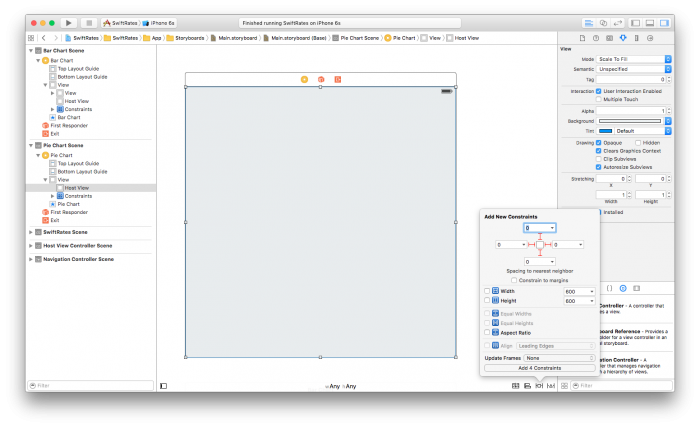Set the background color to any color you like. I used a gray scale color with an opacity of 92%.

Back in PieChartViewController.swift, add the following methods right after `viewDidLoad()`:

```override func viewDidLayoutSubviews() {
super.viewDidLayoutSubviews()
initPlot()
}

func initPlot() {
configureHostView()
configureGraph()
configureChart()
configureLegend()
}

func configureHostView() {
}

func configureGraph() {
}

func configureChart() {
}

func configureLegend() {
}
```

This sets up the plot right after the subviews are laid out. This is the earliest that the `frame` size for the view has been set, which you’ll need to configure the plot.

Each method within `initPlot()` represents a stage in setting up the plot. This helps keep the code a bit more organized.

Add the following to `configureHostView()`:

```hostView.allowPinchScaling = false
```

This disables pinching scaling on the pie chart, which determines whether the host view responds to pinch gestures.

You next need to add a graph to the `hostView`. Add the following to `configureGraph()`:

```// 1 - Create and configure the graph
let graph = CPTXYGraph(frame: hostView.bounds)
hostView.hostedGraph = graph
graph.axisSet = nil

// 2 - Create text style
let textStyle: CPTMutableTextStyle = CPTMutableTextStyle()
textStyle.color = CPTColor.black()
textStyle.fontName = "HelveticaNeue-Bold"
textStyle.fontSize = 16.0
textStyle.textAlignment = .center

// 3 - Set graph title and text style
graph.title = "\(base.name) exchange rates\n\(rate.date)"
graph.titleTextStyle = textStyle
graph.titlePlotAreaFrameAnchor = CPTRectAnchor.top
```

Here’s a breakdown for each section:

1. You first create an instance of `CPTXYGraph` and designate it as the `hostedGraph` of the `hostView`. This associates the graph with the host view.

The `CPTGraph` encompasses everything you see in a standard chart or graph: the border, the title, the plotted data, axes, and legend.

By default, `CPTXYGraph` has a padding of `20` points per side. This doesn’t look great here, so you explicitly set the padding for each side to `0`.

2. You next set up the text style for the graph’s title by creating and configuring a `CPTMutableTextStyle` instance.
3. Lastly, you set the title for the graph and set its style to the one you just created. You also specify the anchoring point to be the top of the view’s bounding rectangle.

Build and run the app, and you should see the chart’s title displayed at the top of the screen: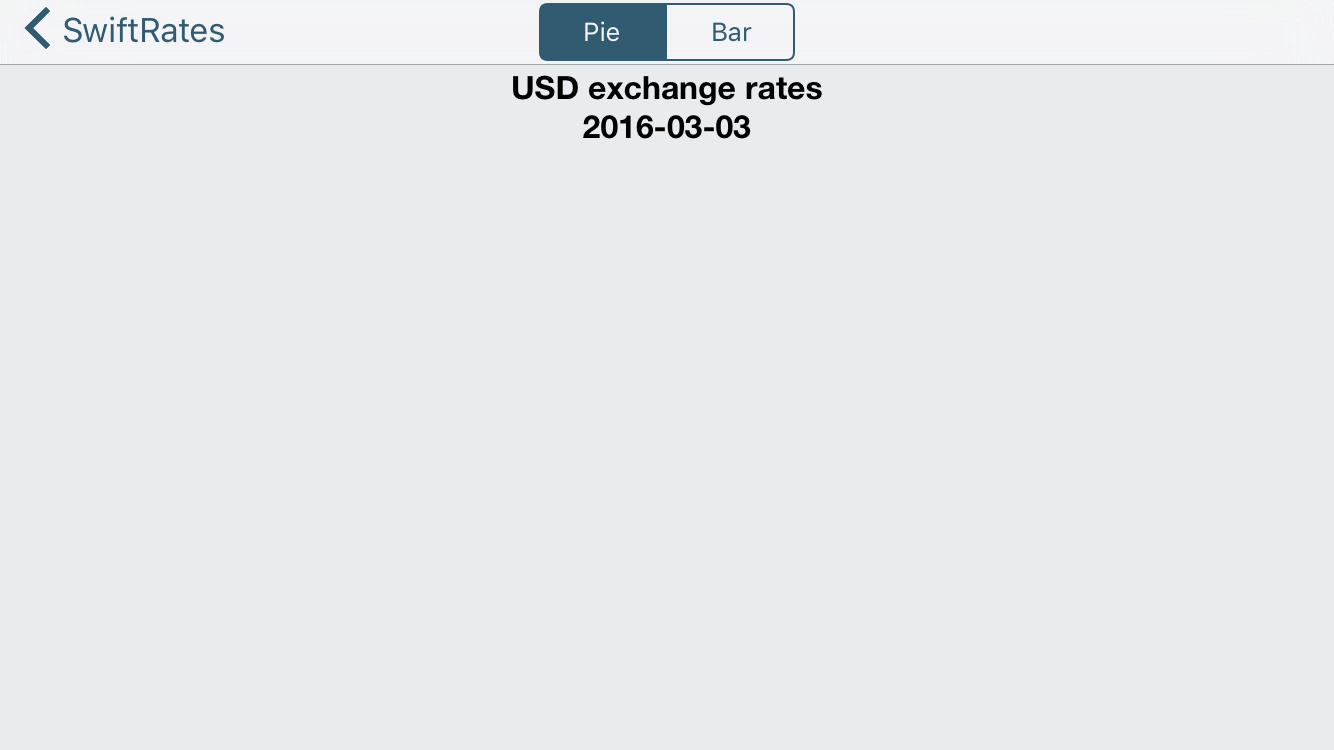### Plotting the Pie Chart

The title looks great, but you know what would be even better in this Core Plot tutorial? Actually seeing the pie chart!

Add the following lines of code to `configureChart()`:

```// 1 - Get a reference to the graph
let graph = hostView.hostedGraph!

// 2 - Create the chart
let pieChart = CPTPieChart()
pieChart.delegate = self
pieChart.dataSource = self
pieChart.pieRadius = (min(hostView.bounds.size.width, hostView.bounds.size.height) * 0.7) / 2
pieChart.identifier = NSString(string: graph.title!)
pieChart.startAngle = CGFloat(M_PI_4)
pieChart.sliceDirection = .clockwise

// 3 - Configure border style
let borderStyle = CPTMutableLineStyle()
borderStyle.lineColor = CPTColor.white()
borderStyle.lineWidth = 2.0
pieChart.borderLineStyle = borderStyle

// 4 - Configure text style
let textStyle = CPTMutableTextStyle()
textStyle.color = CPTColor.white()
textStyle.textAlignment = .center
pieChart.labelTextStyle = textStyle

// 5 - Add chart to graph
```

Here’s what this does:

1. You first get a reference to the graph.
2. You then instantiate a `CPTPieChart`, set its delegate and data source to be the view controller, and configure its appearance.
3. You then configure the chart’s border style.
4. And then, configure its text style.
5. Lastly, you add the pie chart to the graph.

If you build and run the app right now, you’ll see that nothing has changed… This is because you still need to implement the data source and delegate for the pie chart.

First, replace current `numberOfRecords(for:)` with the following:

```func numberOfRecords(for plot: CPTPlot) -> UInt {
return UInt(symbols.count)
}
```

This method determines the number of slices to show on the graph; it will display one pie slice for each symbol.

Next, replace `number(for:field:record:)` with the following:

```func number(for plot: CPTPlot, field fieldEnum: UInt, record idx: UInt) -> Any? {
let symbol = symbols[Int(idx)]
let currencyRate = rate.rates[symbol.name]!.floatValue
return 1.0 / currencyRate
}
```

The pie chart uses this method to get the “gross” value for the currency symbol at the `idx`.

You should note that this value is not a percentage. Rather, this method calculates the currency exchange rate relative to the base currency: the return value of `1.0 / currencyRate` is the exchange rate for “1 base currency per value of another comparison currency.”

`CPTPieChart` will take care of calculating the percentage value for each slice, which ultimately will determine how big each slice is, using these values.

Next, replace `dataLabelForPlot(for:record:)` with the following:

```func dataLabel(for plot: CPTPlot, record idx: UInt) -> CPTLayer? {
let value = rate.rates[symbols[Int(idx)].name]!.floatValue
let layer = CPTTextLayer(text: String(format: "\(symbols[Int(idx)].name)\n%.2f", value))
layer.textStyle = plot.labelTextStyle
return layer
}
```

This method returns a label for the pie slice. The expected return type, `CPTLayer` is similar to a `CALayer`. However, a `CPTLayer` is abstracted to work on both Mac OS X and iOS and provides other drawing niceties used by Core Plot.

Here, you create and return a `CPTTextLayer`, which is a subclass of `CPTLayer` designed to display text.

Finally, you’ll add color to the slices by replacing `sliceFillForPieChart(for:, record:)` with the following:

```func sliceFill(for pieChart: CPTPieChart, record idx: UInt) -> CPTFill? {
switch idx {
case 0:   return CPTFill(color: CPTColor(componentRed:0.92, green:0.28, blue:0.25, alpha:1.00))
case 1:   return CPTFill(color: CPTColor(componentRed:0.06, green:0.80, blue:0.48, alpha:1.00))
case 2:   return CPTFill(color: CPTColor(componentRed:0.22, green:0.33, blue:0.49, alpha:1.00))
default:  return nil
}
}
```

Build and run, and you’ll see a nifty-looking pie chart: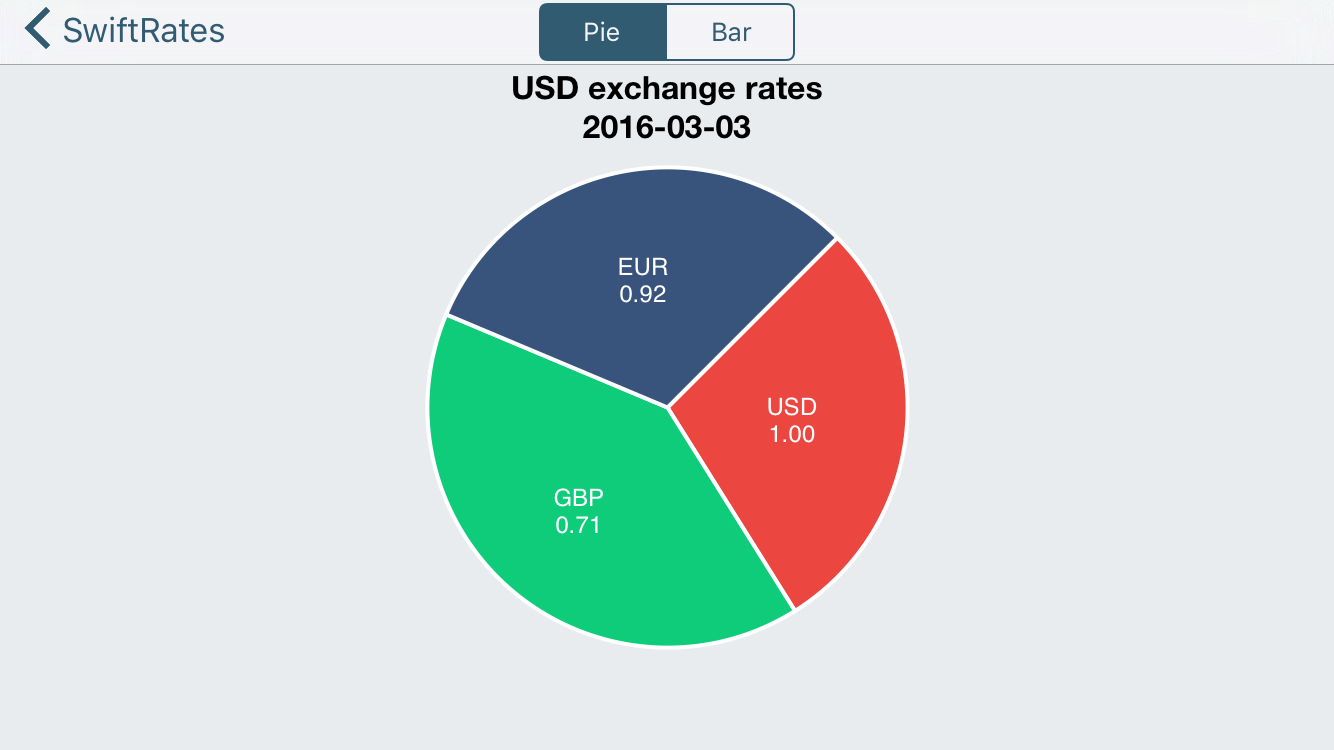### Legen … Wait For It… dary!

The chart looks pretty nice, but adding a legend would make it even better. You’ll now add a legend to the graph in this Core Plot tutorial.

First, replace `configureLegend()` with the following:

```func configureLegend() {
// 1 - Get graph instance
guard let graph = hostView.hostedGraph else { return }

// 2 - Create legend
let theLegend = CPTLegend(graph: graph)

// 3 - Configure legend
theLegend.numberOfColumns = 1
theLegend.fill = CPTFill(color: CPTColor.white())
let textStyle = CPTMutableTextStyle()
textStyle.fontSize = 18
theLegend.textStyle = textStyle

// 4 - Add legend to graph
graph.legend = theLegend
if view.bounds.width > view.bounds.height {
graph.legendAnchor = .right
graph.legendDisplacement = CGPoint(x: -20, y: 0.0)

} else {
graph.legendAnchor = .bottomRight
graph.legendDisplacement = CGPoint(x: -8.0, y: 8.0)
}
}
```

You also need to provide legend data for each slice.

To do this, replace `legendTitleForPieChart(for:record:)` with the following:

```func legendTitle(for pieChart: CPTPieChart, record idx: UInt) -> String? {
return symbols[Int(idx)].name
}
```

Build and run, and you’ll be greeted with a “legendary” graph.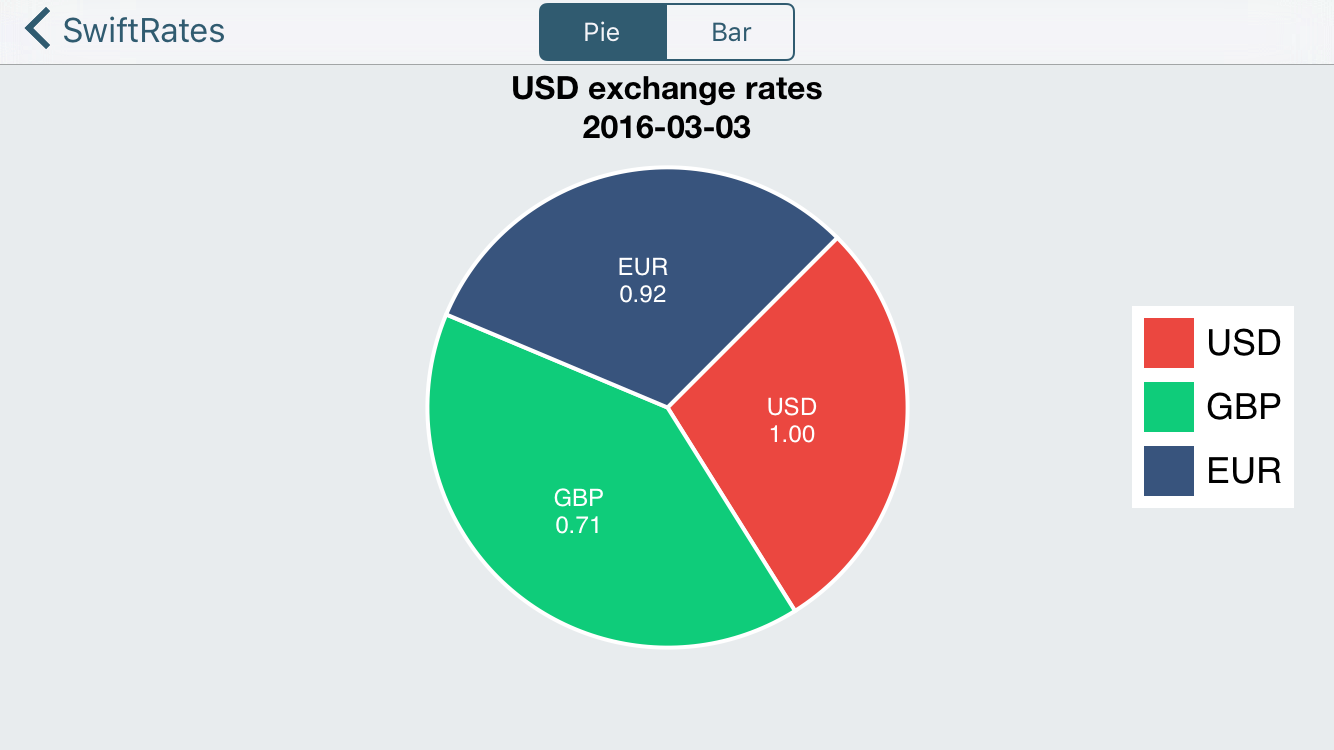## Raising the Bar (Graph)

You’re plotting pie charts like a pro, but it’s time you raised the bar (graph)!

Open `BarGraphViewController` and add the following import:

```import CorePlot
```

```@IBOutlet var hostView: CPTGraphHostingView!
```

Just like a pie chart, the host view will contain the bar graph.

```var plot1: CPTBarPlot!
var plot2: CPTBarPlot!
var plot3: CPTBarPlot!
```

Here you declare three `CPTBarPlot` properties, which will correspond to each currency shown on the graph.

Note there are also three `IBOutlet` labels and three `IBAction` methods already defined, all of which have already been connected for you on the storyboard.

Lastly, add the following extension at the end of the file:

```extension BarGraphViewController: CPTBarPlotDataSource, CPTBarPlotDelegate {

func numberOfRecords(for plot: CPTPlot) -> UInt {
return 0
}

func number(for plot: CPTPlot, field fieldEnum: UInt, record idx: UInt) -> Any? {
return 0
}

func barPlot(_ plot: CPTBarPlot, barWasSelectedAtRecord idx: UInt, with event: UIEvent) {

}
}
```

This too is similar to a pie chart: you provide the data for a bar chart via `CPTBarPlotDataSource`, and you get user interaction events via `CPTBarPlotDelegate`. You’ll write these in a bit.

### Setting Up the Graph Host View (again!)

Again, just like you did for the pie chart in this Core Plot tutorial, you need to add the host view via Interface Builder.

Return to Main.storyboard, and select the `BarGraphViewController` scene.

Drag a new `UIView` onto the view; change its class to `CPTGraphHostingView`; and connect its outlet to the `hostView` on the controller.

Update its frame to the following via the Utilities\Size Inspector (the ruler tab):

X = 0, Y = 53, Width = 600, Height = 547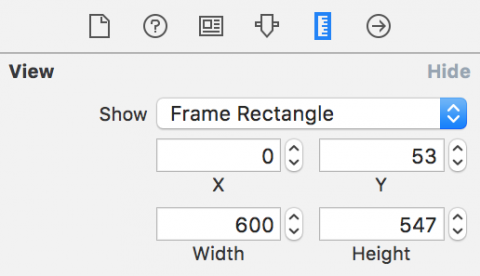Add constraints to pin it to all of its neighbors, making sure that Constrain to margins is NOT set.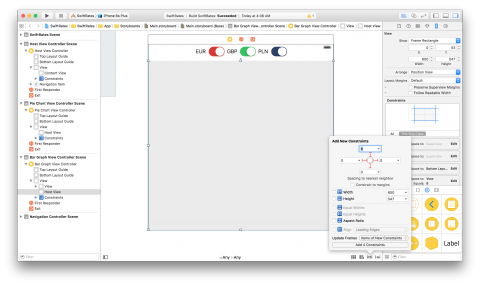Lastly, set the background color to any color you like. Again, I used a gray scale color with an opacity of 92%.

### Plotting the Bar Graph

Now that the UI is all hooked up in this Core Plot tutorial, it’s time to plot the bar graph.

First, back in `BarGraphViewController`, you need a couple constant properties. Add the following right below the other properties:

```let BarWidth = 0.25
let BarInitialX = 0.25
```

You’re also going to need a helper function to calculate the highest rate value. Add the following function right after `updateLabels()`:

```func highestRateValue() -> Double {
var maxRate = DBL_MIN
for rate in rates {
maxRate = max(maxRate, rate.maxRate().doubleValue)
}
return maxRate
}
```

Next, add the following methods, right after `highestRateValue()`:

```override func viewDidLayoutSubviews() {
super.viewDidLayoutSubviews()
initPlot()
}

func initPlot() {
configureHostView()
configureGraph()
configureChart()
configureAxes()
}

func configureHostView() {
}

func configureGraph() {
}

func configureChart() {
}

func configureAxes() {
}
```

Does this look familiar? Yep, it’s nearly the exact same structure as before.

Add the following to `configureHostView()`:

```hostView.allowPinchScaling = false
```

Again, as you won’t be using pinch scaling, you should disable it.

Next, add the following lines to `configureGraph()`:

```// 1 - Create the graph
let graph = CPTXYGraph(frame: hostView.bounds)
hostView.hostedGraph = graph

// 2 - Configure the graph
graph.apply(CPTTheme(named: CPTThemeName.plainWhiteTheme))
graph.fill = CPTFill(color: CPTColor.clear())

// 3 - Set up styles
let titleStyle = CPTMutableTextStyle()
titleStyle.color = CPTColor.black()
titleStyle.fontName = "HelveticaNeue-Bold"
titleStyle.fontSize = 16.0
titleStyle.textAlignment = .center
graph.titleTextStyle = titleStyle

let title = "\(base.name) exchange rates\n\(rates.first!.date) - \(rates.last!.date)"
graph.title = title
graph.titlePlotAreaFrameAnchor = .top
graph.titleDisplacement = CGPoint(x: 0.0, y: -16.0)

// 4 - Set up plot space
let xMin = 0.0
let xMax = Double(rates.count)
let yMin = 0.0
let yMax = 1.4 * highestRateValue()
guard let plotSpace = graph.defaultPlotSpace as? CPTXYPlotSpace else { return }
plotSpace.xRange = CPTPlotRange(locationDecimal: CPTDecimalFromDouble(xMin), lengthDecimal: CPTDecimalFromDouble(xMax - xMin))
plotSpace.yRange = CPTPlotRange(locationDecimal: CPTDecimalFromDouble(yMin), lengthDecimal: CPTDecimalFromDouble(yMax - yMin))
```

Here’s a break down of what’s happening:

1. First, you instantiate a `CPTXYGraph`, which is essentially a bar graph, and associate it with the `hostView`.
2. You then declare the default theme to be plain white and set the padding on the left and bottom to allow room for axes.
3. You next setup the text style, set the chart’s title, and the title’s position.
4. Lastly, you configure the `CPTXYPlotSpace`, which is responsible for mapping device coordinates to the coordinates of your graph.

For this graph, you’re plotting three exchange rates that use the same plot space. However, it’s also possible to have a separate plot space for each plot.

You also use an assumed minimum and maximum exchange rate range on the plot space. Later in the Core Plot tutorial, you’ll see how you can auto-size the plot space when you don’t know the range in advance.

Now that you have your graph, it’s time to add some plots to it! Add the following code to `configureChart()`:

```// 1 - Set up the three plots
plot1 = CPTBarPlot()
plot1.fill = CPTFill(color: CPTColor(componentRed:0.92, green:0.28, blue:0.25, alpha:1.00))
plot2 = CPTBarPlot()
plot2.fill = CPTFill(color: CPTColor(componentRed:0.06, green:0.80, blue:0.48, alpha:1.00))
plot3 = CPTBarPlot()
plot3.fill = CPTFill(color: CPTColor(componentRed:0.22, green:0.33, blue:0.49, alpha:1.00))

// 2 - Set up line style
let barLineStyle = CPTMutableLineStyle()
barLineStyle.lineColor = CPTColor.lightGray()
barLineStyle.lineWidth = 0.5

// 3 - Add plots to graph
guard let graph = hostView.hostedGraph else { return }
var barX = BarInitialX
let plots = [plot1!, plot2!, plot3!]
for plot: CPTBarPlot in plots {
plot.dataSource = self
plot.delegate = self
plot.barWidth = NSNumber(value: BarWidth)
plot.barOffset = NSNumber(value: barX)
plot.lineStyle = barLineStyle
barX += BarWidth
}
```

Here’s what the above code does:

1. You instantiate each bar plot and set each one’s fill color.
2. You instantiate a `CPTMutableLineStyle` instance that represents the outer border of each bar plot.
3. You apply a “common configuration” to each bar plot. This configuration includes setting the data source and delegate, setting the width and relative (left-right) placement of each bar in the plot, setting the line style, and finally, adding the plot to the graph.

While you still won’t be able to see the bar graph in action yet, build the app to verify everything compiles correctly so far.

In order to actually display the data on the bar graph, you need to implement the delegate methods that provide the necessary data to the graph.

Replace `numberOfRecordsForPlot(for:)` with the following:

```return UInt(rates.count)
```

This method returns the number of records that should be displayed.

Replace `numberForPlot(for:field:record:)` with the following:

```
if fieldEnum == UInt(CPTBarPlotField.barTip.rawValue) {
if plot == plot1 {
return 1.0 as AnyObject?
}
if plot == plot2 {
return rates[Int(idx)].rates[symbols.name]!
}
if plot == plot3 {
return rates[Int(idx)].rates[symbols.name]!
}
}
return idx
```

The `CPTBarPlotField.BarTip` value indicates the relative size of the bar chart. You use the retained properties to figure out the exchange rate for which you need to retrieve the data. The `idx` corresponds to the index of the rate of interest.

Build and run, and you should see something similar to the following: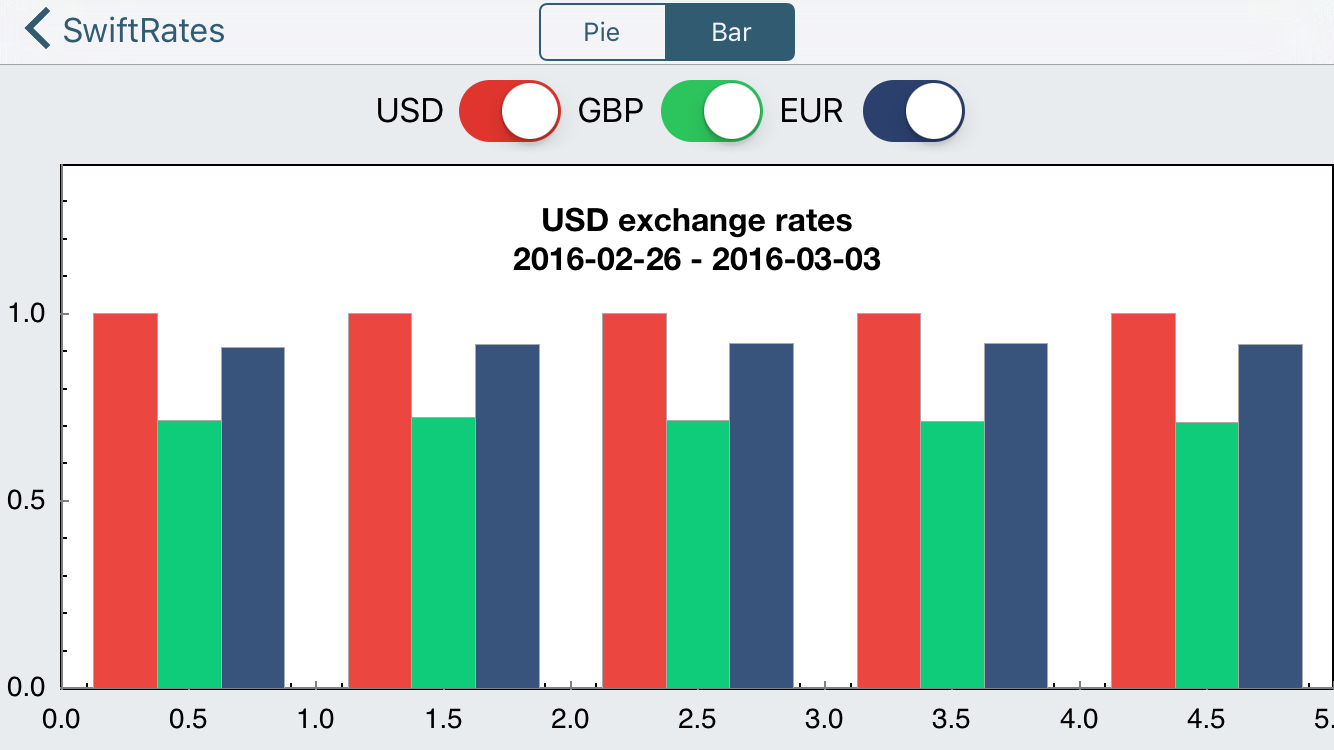You’re getting there! However, notice isn’t any indication of what each axis represents.

To fix this, add the following to `configureAxes()`:

```// 1 - Configure styles
let axisLineStyle = CPTMutableLineStyle()
axisLineStyle.lineWidth = 2.0
axisLineStyle.lineColor = CPTColor.black()
// 2 - Get the graph's axis set
guard let axisSet = hostView.hostedGraph?.axisSet as? CPTXYAxisSet else { return }
// 3 - Configure the x-axis
if let xAxis = axisSet.xAxis {
xAxis.labelingPolicy = .none
xAxis.majorIntervalLength = 1
xAxis.axisLineStyle = axisLineStyle
var majorTickLocations = Set<NSNumber>()
var axisLabels = Set<CPTAxisLabel>()
for (idx, rate) in rates.enumerated() {
majorTickLocations.insert(NSNumber(value: idx))
let label = CPTAxisLabel(text: "\(rate.date)", textStyle: CPTTextStyle())
label.tickLocation = NSNumber(value: idx)
label.offset = 5.0
label.alignment = .left
axisLabels.insert(label)
}
xAxis.majorTickLocations = majorTickLocations
xAxis.axisLabels = axisLabels
}
// 4 - Configure the y-axis
if let yAxis = axisSet.yAxis {
yAxis.labelingPolicy = .fixedInterval
yAxis.labelOffset = -10.0
yAxis.minorTicksPerInterval = 3
yAxis.majorTickLength = 30
let majorTickLineStyle = CPTMutableLineStyle()
majorTickLineStyle.lineColor = CPTColor.black().withAlphaComponent(0.1)
yAxis.majorTickLineStyle = majorTickLineStyle
yAxis.minorTickLength = 20
let minorTickLineStyle = CPTMutableLineStyle()
minorTickLineStyle.lineColor = CPTColor.black().withAlphaComponent(0.05)
yAxis.minorTickLineStyle = minorTickLineStyle
yAxis.axisLineStyle = axisLineStyle
}
```

Simply put, the above code first defines styles for the axis lines and titles. Then, the code retrieves the axis set for the graph and configures the settings for the x and y axes.

Build and run to see the result of this change.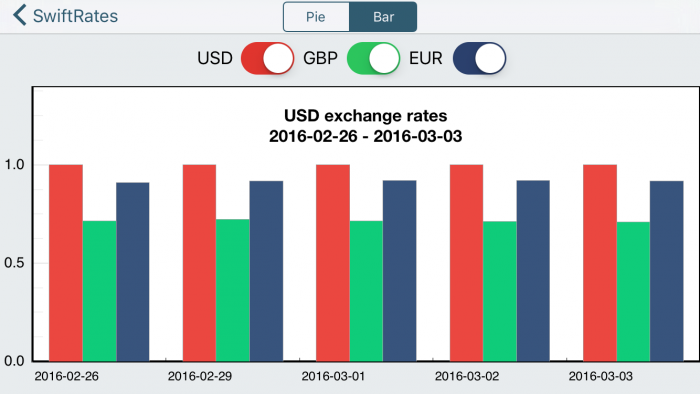### Grinding Axes

Much better, right? The only drawback is that your axes are plain – giving no idea of the exact exchange rate.

You can fix this so that when a user taps on an individual bar chart, the app will display the price that the bar represents. To do this, add a new property:

```var priceAnnotation: CPTPlotSpaceAnnotation?
```

Then add the following code to `barPlot(for:barWasSelectedAtRecord:with:)`:

```// 1 - Is the plot hidden?
if plot.isHidden == true {
return
}
// 2 - Create style, if necessary
let style = CPTMutableTextStyle()
style.fontSize = 12.0
style.fontName = "HelveticaNeue-Bold"

// 3 - Create annotation
guard let price = number(for: plot,
field: UInt(CPTBarPlotField.barTip.rawValue),
record: idx) as? CGFloat else { return }

priceAnnotation?.annotationHostLayer?.removeAnnotation(priceAnnotation)
priceAnnotation = CPTPlotSpaceAnnotation(plotSpace: plot.plotSpace!, anchorPlotPoint: [0,0])

// 4 - Create number formatter
let formatter = NumberFormatter()
formatter.maximumFractionDigits = 2
// 5 - Create text layer for annotation
let priceValue = formatter.string(from: NSNumber(cgFloat: price))
let textLayer = CPTTextLayer(text: priceValue, style: style)

priceAnnotation!.contentLayer = textLayer
// 6 - Get plot index
var plotIndex: Int = 0
if plot == plot1 {
plotIndex = 0
}
else if plot == plot2 {
plotIndex = 1
}
else if plot == plot3 {
plotIndex = 2
}
// 7 - Get the anchor point for annotation
let x = CGFloat(idx) + CGFloat(BarInitialX) + (CGFloat(plotIndex) * CGFloat(BarWidth))
let y = CGFloat(price) + 0.05
priceAnnotation!.anchorPlotPoint = [NSNumber(cgFloat: x), NSNumber(cgFloat: y)]
// 8 - Add the annotation
guard let plotArea = plot.graph?.plotAreaFrame?.plotArea else { return }
}
```

This requires a bit of explanation:

1. You don’t display an annotation for a hidden plot. While the plots currently don’t have the ability to be hidden, you’ll be implementing this in the next step when you integrate the switches with the chart.
2. Here you create a text style for your annotation.
3. You then get the price for the specified plot and then create an annotation object if one doesn’t exist.
4. You create a number formatter if one doesn’t exist, since you’ll need to format the price for display.
5. You create a text layer using the formatted price, and set the content layer for the annotation to this new text layer.
6. You get the plot index for the plot for which you’ll display the annotation.
7. You calculate the annotation position based on the plot index, and then set the `anchorPlotPoint` for the annotation using the calculated position.
8. Finally, you add the annotation to the graph.

Build and run. Every time you tap on a bar in your chart, the value for that bar should pop up right above the bar.

Nifty! :]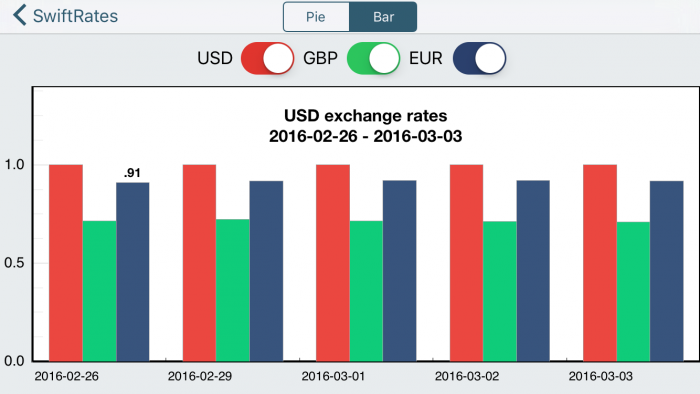### Hide and Seek

The bar graph looks great, but the switches at the top of the screen do nothing. It’s time in this Core Plot tutorial to rectify that.

First, you’ll need to add a helper method. Add the following right after `switch3Changed(_:)`:

```func hideAnnotation(graph: CPTGraph) {
guard let plotArea = graph.plotAreaFrame?.plotArea,
let priceAnnotation = priceAnnotation else {
return
}
plotArea.removeAnnotation(priceAnnotation)
self.priceAnnotation = nil
}
```

The code simply removes an annotation, if it exists.

Next, you want the user to be able to toggle the display of bar charts for a given currency using the switches.

To do such, replace the implementations for `switch1Changed(_:)`, `switch2Changed(_:)`, and `switch3Changed(_:)` with the following:

```@IBAction func switch1Changed(_ sender: UISwitch) {
let on = sender.isOn
if !on {
hideAnnotation(graph: plot1.graph!)
}
plot1.isHidden = !on
}

@IBAction func switch2Changed(_ sender: UISwitch) {
let on = sender.isOn
if !on {
hideAnnotation(graph: plot2.graph!)
}
plot2.isHidden = !on
}

@IBAction func switch3Changed(_ sender: UISwitch) {
let on = sender.isOn
if !on {
hideAnnotation(graph: plot3.graph!)
}
plot3.isHidden = !on
}
```

The logic is fairly simple. If the switch is set to off, the corresponding plot and any visible annotation is hidden. If the switch is set to on, then the plot is made visible again.

Build and run. You can now toggle each bar chart to your heart’s content. Nice work on this Core Plot tutorial!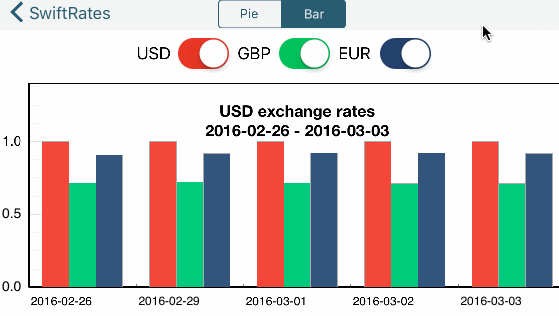## Where to Go From Here?

Whew- that was fun! Hopefully, this Core Plot tutorial underscores the power of Core Plot and gives you ideas for how it can be used within your own apps.

Be sure to refer to the Core Plot repo for more information, including documentation, examples, and tips.

Happy plotting!

5/5

2 ratings